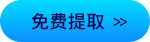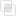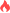python中使用动量交易策略2021-04-28 15:19:47903浏览 · 0收藏 · 0评论1、说明

2、实例

```# 导入相关模块
import numpy as np
import tushare as ts
import pandas as pd
import mplfinance as mpf
import matplotlib.pyplot as plt

token = 'Your token'   # 输入你的接口密匙，获取方式及相关权限见Tushare官网。
pro = ts.pro_api(token)
df = pro.daily(ts_code='000001.SZ')  # daily为tushare的股票数据接口。

# 将获取到的DataFrame数据进行标准化处理，转换为方便自己使用的一种规范格式。
df = df.loc[:, ['trade_date', 'open', 'high', 'low', 'close', 'vol']]
df.rename(
columns={
'high': 'High', 'low': 'Low',
'close': 'Close', 'vol': 'Volume'},
inplace=True)       # 重定义列名，方便统一规范操作。
df['Date'] = pd.to_datetime(df['Date'])  # 转换日期列的格式，便于作图
df.set_index(['Date'], inplace=True)  # 将日期列作为行索引
df = df.sort_index()  # 倒序，因为Tushare的数据是最近的交易日数据显示在DataFrame上方，倒序后方能保证作图时X轴从左到右时间序列递增。```python如何实现事务机制Python bs4的四种对象Python运行过程的分析Python中__slots__限制属性Python测试前置操作的方法Python unittest有哪些使用方法python动态规划算法的使用过程python GIL的使用及弊端处理python线程安全的介绍及解决方法python线程池的四种好处归纳python初学者爬虫的学习方向python迭代器协议支持的两种方法python生成器表达式如何节省内存java循环和递归的区别320

java枚举如何实现单例382

java中final域的内存语义理解713